2020 NBA Defensive Player of the Year Odds
+200
2 to 1Rudy Gobert
21.2% implied probability

+250
2.5 to 1Myles Turner
18.2% implied probability

+300
3 to 1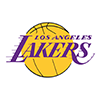Anthony Davis
15.9% implied probability

+700
7 to 1Giannis Antetokounmpo
8.0% implied probability

+1000
10 to 1Ben Simmons
5.8% implied probability

No changes have been recorded yet.
+1200
12 to 1Joel Embiid
4.9% implied probability

+2000
20 to 1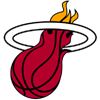Bam Adebayo
3.0% implied probability

+4000
40 to 1Draymond Green
1.6% implied probability

+6000
60 to 1Kawhi Leonard
1.0% implied probability

+8000
80 to 1Marcus Smart
0.8% implied probability

+10000
100 to 1Kevin Durant
0.6% implied probability

+10000
100 to 1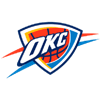Luguentz Dort
0.6% implied probability

+10000
100 to 1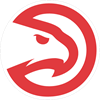Clint Capela
0.6% implied probability

No changes have been recorded yet.
+15000
150 to 1Andre Drummond
0.4% implied probability

+15000
150 to 1Robert Covington
0.4% implied probability

+15000
150 to 1Steven Adams
0.4% implied probability

No changes have been recorded yet.
+15000
150 to 1Jaylen Brown
0.4% implied probability

+15000
150 to 1Pascal Siakam
0.4% implied probability

+15000
150 to 1Brook Lopez
0.4% implied probability

+15000
150 to 1Jimmy Butler
0.4% implied probability

+15000
150 to 1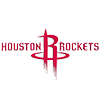P.J. Tucker
0.4% implied probability

No changes have been recorded yet.
+15000
150 to 1Jayson Tatum
0.4% implied probability

+15000
150 to 1OG Anunoby
0.4% implied probability

+15000
150 to 1Paul George
0.4% implied probability

+15000
150 to 1Eric Bledsoe
0.4% implied probability

+15000
150 to 1Jarrett Allen
0.4% implied probability

+15000
150 to 1Patrick Beverley
0.4% implied probability

+15000
150 to 1Kristaps Porzingis
0.4% implied probability

+15000
150 to 1Jrue Holiday
0.4% implied probability

+15000
150 to 1Deandre Ayton
0.4% implied probability

No changes have been recorded yet.
+15000
150 to 1Mitchell Robinson
0.4% implied probability

+15000
150 to 1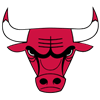Wendell Carter
0.4% implied probability

No changes have been recorded yet.
+25000
250 to 1Karl-Anthony Towns
0.3% implied probability

No changes have been recorded yet.
+25000
250 to 1Cameron Payne
0.3% implied probability

No changes have been recorded yet.
+25000
250 to 1Josh Richardson
0.3% implied probability

+25000
250 to 1Matisse Thybulle
0.3% implied probability

+25000
250 to 1Maxi Kleber
0.3% implied probability

No changes have been recorded yet.
+25000
250 to 1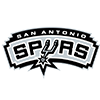Dejounte Murray
0.3% implied probability

No changes have been recorded yet.
+25000
250 to 1Ivica Zubac
0.3% implied probability

No changes have been recorded yet.
+25000
250 to 1Fred VanVleet
0.3% implied probability

No changes have been recorded yet.
+25000
250 to 1Zion Williamson
0.3% implied probability

No changes have been recorded yet.
+25000
250 to 1Otto Porter
0.3% implied probability

No changes have been recorded yet.
+25000
250 to 1Alex Caruso
0.3% implied probability

No changes have been recorded yet.
+25000
250 to 1Kyle Kuzma
0.3% implied probability

No changes have been recorded yet.
+25000
250 to 1Pat Connaughton
0.3% implied probability

No changes have been recorded yet.
+25000
250 to 1LeBron James
0.3% implied probability

+25000
250 to 1Montrezl Harrell
0.3% implied probability

No changes have been recorded yet.
+25000
250 to 1Avery Bradley
0.3% implied probability

+25000
250 to 1Paul Millsap
0.3% implied probability

No changes have been recorded yet.
+25000
250 to 1Kyle Lowry
0.3% implied probability

+25000
250 to 1Marc Gasol
0.3% implied probability

+25000
250 to 1DeAndre Jordan
0.3% implied probability

No changes have been recorded yet.
+25000
250 to 1George Hill
0.3% implied probability

No changes have been recorded yet.
+25000
250 to 1Serge Ibaka
0.3% implied probability

+25000
250 to 1Danny Green
0.3% implied probability

+25000
250 to 1Nikola Jokic
0.3% implied probability

No changes have been recorded yet.
+25000
250 to 1Donte DiVincenzo
0.3% implied probability

No changes have been recorded yet.
+25000
250 to 1Nerlens Noel
0.3% implied probability

No changes have been recorded yet.
+25000
250 to 1Gary Harris
0.3% implied probability

+25000
250 to 1Chris Paul
0.3% implied probability

No changes have been recorded yet.
+25000
250 to 1Khris Middleton
0.3% implied probability

+25000
250 to 1Daniel Theis
0.3% implied probability

No changes have been recorded yet.
+25000
250 to 1Gorgui Dieng
0.3% implied probability

No changes have been recorded yet.
+25000
250 to 1Victor Oladipo
0.3% implied probability

No changes have been recorded yet.
+50000
500 to 1Enes Kanter
0.1% implied probability

No changes have been recorded yet.
+50000
500 to 1Dennis Schroder
0.1% implied probability

No changes have been recorded yet.
+50000
500 to 1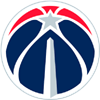Russell Westbrook
0.1% implied probability

No changes have been recorded yet.
+50000
500 to 1Stephen Curry
0.1% implied probability

No changes have been recorded yet.
+50000
500 to 1Kyrie Irving
0.1% implied probability

No changes have been recorded yet.
+50000
500 to 1Luka Doncic
0.1% implied probability

No changes have been recorded yet.
+50000
500 to 1James Harden
0.1% implied probability

No changes have been recorded yet.
+50000
500 to 1Trae Young
0.1% implied probability

No changes have been recorded yet.
+50000
500 to 1Damian Lillard
0.1% implied probability

No changes have been recorded yet.
+50000
500 to 1Gordon Hayward
0.1% implied probability

No changes have been recorded yet.
+50000
500 to 1Nikola Vucevic
0.1% implied probability

No changes have been recorded yet.
+50000
500 to 1Donovan Mitchell
0.1% implied probability

No changes have been recorded yet.